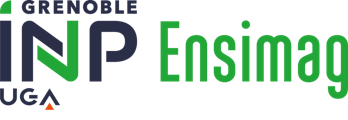Une voie, plusieurs choix
Informatique et Mathématiques appliquées# Advanced algorithms approximation parallel and randomized algorithms - Complexity - 4MMALGOA

A+Augmenter la taille du texteA-Réduire la taille du texteImprimer le documentEnvoyer cette page par mail cet article Facebook Twitter Linked In
• #### Number of hours

• Lectures : 13.5
• Tutorials : 13.5
• Laboratory works : 6.0
ECTS : 2.5

### Goals

This course presents the complexity theory (lower bounds, complexity classes and reduction, NP classification) and presents the main techniques to design efficient algorithms: parallel, randomized and approximation algorithms, heuristics.
The course is coupled to a practical project on the programming of a multi-players game in C++.

Contact Jean-Louis ROCH

Content

1. Machine models, Problem Complexity. Heuristics (e.g. alpha-beta)
2. Complexity; lower bounds; reduction.
3. Complexity classes P, NP, NP-complete.
4. Approximation algorithms. APX classes.
5. Randomized Algorithms. [BRZ]PP classes.
6. Parallel Algorithms. [ RZ][AN]C classes.
7. Interactive algorithms and proofs (IP). Polynomial hierarchy.

Prerequisites

Very good understanding of cost analysis for simple sequential algorithms (iterative / loops, recursive / divide and conquer).
Prerequisites: Algorithms and programming (1st year, 3MMALG2), elementary notions on graphs (3MMRO) and on computation models (3MMTL2).

Tests

One individual written examination (2h)(E: coef 3 / 4). One programming work with report (P: coef 1 / 4).

N1=3/4E1+1/4P
N2=3/4E2+1/4P

Curriculum->MMIS.->Semester 4
Curriculum->ENGINEERING systm of information->Semester 4
Curriculum->For Financial Engineering->Semester 4

Bibliography

S. Arora, B. Barak, Computaional complexity: a modern approach, 2009
P. Berlioux, M.-P. Cani, A. Lux, R. Mohr, D. Naddef, J.-L. Roch : Algorithmique II.. Polycopié ENSIMAG
T. Cormen, C.E. Leiserson, R. Rivest, C. Stein : Introduction to Algorithms, 2nd edition, 2001

A+Augmenter la taille du texteA-Réduire la taille du texteImprimer le documentEnvoyer cette page par mail cet article Facebook Twitter Linked InÉcole nationale supérieure d'informatique et de mathématiques appliquées
681, rue de la passerelle - Domaine universitaire - BP 72
38402 SAINT MARTIN D'HERES